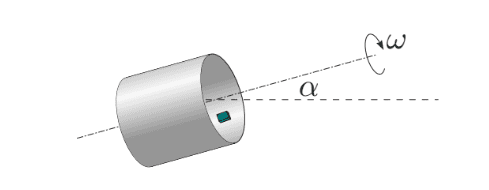# Cylinder rolling with a block inside it

Homework Statement:
A cylinder with radius R spins around its axis with an
angular speed ω. On its inner surface there lies a small block;

the coefficient of friction between the block and the inner sur-
face of the cylinder is μ. Find the values of ω for which the

block does not slip (stays still with respect to the cylinder).

Consider the cases where (a) the axis of the cylinder is hori-
zontal; (b) the axis is inclined by angle α with respect to the

horizon.
Relevant Equations:
so I as it is in rotation
it will experience centripetal force
and required angle for a object not to slip = tanμ
so I expressed cos and sin in it and got this relation
mω²rμ +mg(μ/√μ²+1) =mg(μ/√μ²+1)
but this is irrelevant for finding ω .Last edited by a moderator:

Lnewqban
Gold Member
We need the small block to remain where it is respect to the rotating cylinder.
Taking the ground as reference: the magnitude of the force associate to rotation remains constant, but its direction constantly changes, while the force associate to gravity always points downwards.
We need to find out the worst condition (both forces oppose each other), making the cylinder spin fast enough as to keep rotation force being at least slightly greater than gravity force for that worst condition.
That applies to each degree of tilting of the cylinder ( (a) the axis of the cylinder is hori-
zontal; (b) the axis is inclined by angle α with respect to the horizon).

We need the small block to remain where it is respect to the rotating cylinder.
Taking the ground as reference: the magnitude of the force associate to rotation remains constant, but its direction constantly changes, while the force associate to gravity always points downwards.
We need to find out the worst condition (both forces oppose each other), making the cylinder spin fast enough as to keep rotation force being at least slightly greater than gravity force for that worst condition.
can I take rotating frame of reference??

•Lnewqban
can I take rotating frame of reference??
but doing so will change the direction of gravity respect to cylinder i think.

Lnewqban
Gold Member
can I take rotating frame of reference??
Of course you can.
But remember, we only need to study the worst situations for both cases (analogy: top inverted position of a car in a roller coster).

Of course you can.
But remember, we only need to study the worst situations for both cases.
wont the direction of gravity be changing constantly?

•Lnewqban
Lnewqban
Gold Member
wont the direction of gravity be changing constantly?
Yes, but sometimes it will help the small block to stay put, sometimes it will not.